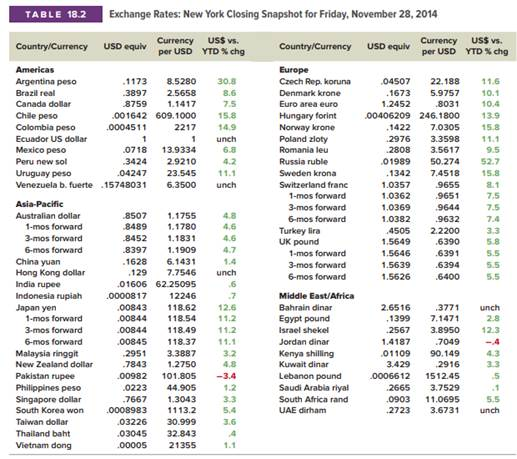### Create an Account

Home / Questions / Interest Rate Parity Use Table 182 to answer the following questions Suppose interest rate...

# Interest Rate Parity Use Table 182 to answer the following questions Suppose interest rate parity holds and the current riskfree rate in the United States is 12 percent per six months What must

Interest Rate Parity. Use Table 18.2 to answer the following questions. Suppose interest rate parity holds, and the current risk-free rate in the United States is 1.2 percent per six months. What must the six-month risk-free rate be in Australia? In Japan? In Great Britain?May 25 2020 View more View LessSubscribe To Get Solution